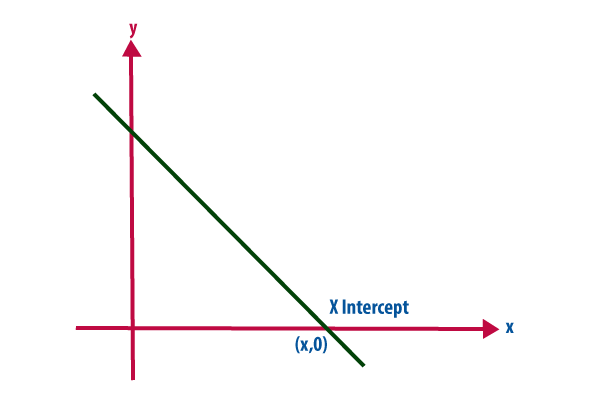# X Intercept Formula

In analytic geometry, using the common convention that the horizontal axis represents a variable x and the vertical axis represents a variable y and an x-intercept is a point where the graph of a function or relation intersects with the x-axis of the coordinate system.The X-Intercept Formula of a line Ax + By = C is,

$\large x=\frac{C}{A}$

The X-Intercept Formula of a line in the slope-intercept form y = mx + c is,

$\large x=\frac{-c}{m}$

Where,
m is the slope of the line.
c is the intercept of the line.

The X-Intercept Formula of a line in the point-slope form y – b = m(x – a) is,

$\large x=\frac{-b}{m}+a$

Where,
m is the slope of the line.
(a, b) is a point on the line.

### Solved Example

Example: Find the X-Intercept of the equation 3x + 4y = 12.

Solution:
To find the x-intercept, set y = 0 and solve for x.

$$\begin{array}{l}3x+4(0)=12\end{array}$$
$$\begin{array}{l}3x+0=12\end{array}$$
$$\begin{array}{l}3x=12\end{array}$$
$$\begin{array}{l}x=\frac{12}{3}\end{array}$$
$$\begin{array}{l}x=4\end{array}$$

Alternatively,

Comaring the given equation with Ax + By = C,

A = 3, B = 4 and C = 12

x-intercept = C/A = 12/3 = 4